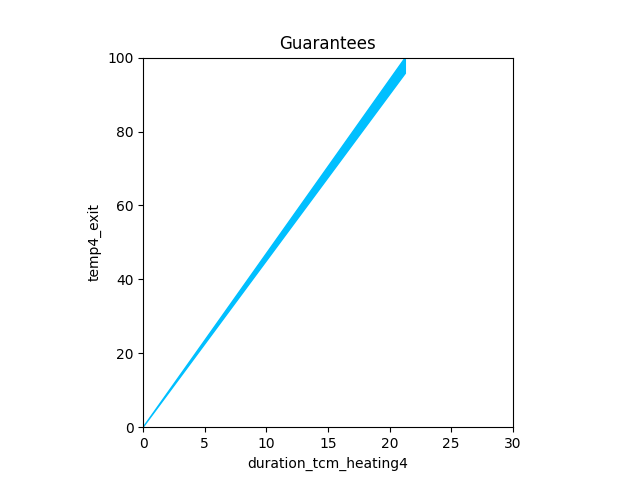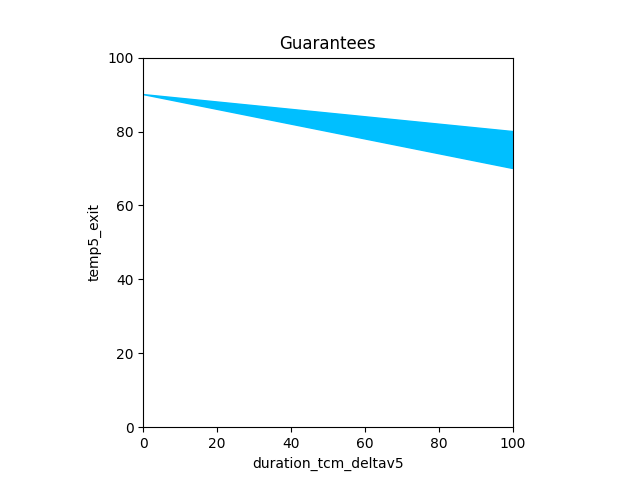# Thermal Viewpoint

%matplotlib widget

from pacti import write_contracts_to_file
from pacti.terms.polyhedra import *
from pacti.iocontract import Var
from pacti.contracts import PolyhedralIoContract
from contract_utils import *
from plot_utils import plot_guarantees_with_bounds_hover


## Thermal viewpoint modeling¶

Except for TCM, all other tasks have the same qualitative thermal impact: the spacecraft's thrusters quickly reach their coldest temperature since they are off and have no thermal insulation or significant thermal capacity. Consequently, we define a common contract template for modeling the cooling impact in a way that is independent of the scheduled task duration.

def COOLING_thermal(s: int) -> PolyhedralIoContract:
spec = PolyhedralIoContract.from_strings(
input_vars=[
f"temp{s}_entry",   # initial thrusters temperature
],
output_vars=[
f"temp{s}_exit",    # final thrusters temperature
],
assumptions=[
# Upper-bound thruster temperature
f"temp{s}_entry <= 100",
# lower-bound thruster temperature
f"-temp{s}_entry <= 0",
],
guarantees=[
# thrusters quickly cool down to their lowest temperature
f" temp{s}_exit = 0",
],
)
return spec


Objective: charge the spacecraft battery

As summarized in the qualitative impacts table, this function affects this viewpoint according to the cooling impact defined above.

charging1_thermal = COOLING_thermal(s=2)
print(f"Contract charging1_thermal:\n\n{charging1_thermal}")

Contract charging1_thermal:

InVars: [temp2_entry]
OutVars:[temp2_exit]
A: [
temp2_entry <= 100
-temp2_entry <= -0
]
G: [
temp2_exit = 0
]


Objective: downlink science data to Earth.

As summarized in the qualitative impacts table, this function affects this viewpoint according to the cooling impact defined above.

dsn1_thermal = COOLING_thermal(s=1)
print(f"Contract dsn1_thermal:\n\n{dsn1_thermal}")

Contract dsn1_thermal:

InVars: [temp1_entry]
OutVars:[temp1_exit]
A: [
temp1_entry <= 100
-temp1_entry <= -0
]
G: [
temp1_exit = 0
]


### SBO Task (Small body observations)¶

Objective: Acquire small body observations (science data & navigation)

As summarized in the qualitative impacts table, this function affects this viewpoint according to the cooling impact defined above.

sbo1_thermal = COOLING_thermal(s=3)
print(f"Contract sbo1_thermal:\n\n{sbo1_thermal}")

Contract sbo1_thermal:

InVars: [temp3_entry]
OutVars:[temp3_exit]
A: [
temp3_entry <= 100
-temp3_entry <= -0
]
G: [
temp3_exit = 0
]


### TCM Task (Perform a Trajectory Correction Maneuver)¶

Objective: Perform a delta-V maneuver to bring the spacecraft trajectory closer to that of the small body.

As summarized in the qualitative impacts table, this function affects this viewpoint with impacts that are linear with the duration of the task:

• Thermal:
• the thrusters must be heated before firing them, thereby discharging the battery proportionally to a consumption rate.
• the deltaV maneuver fires the spacecraft thrusters, keeping them from cooling.

def TCM_heating_thermal(s: int, heating_rate: Tuple[float, float]) -> PolyhedralIoContract:
spec = PolyhedralIoContract.from_strings(
input_vars=[
f"temp{s}_entry",           # initial thrusters temperature
f"duration_tcm_heating{s}", # allocation variable for TCM heating duration
],
output_vars=[
f"temp{s}_exit",            # final thrusters temperature
],
assumptions=[
# Task has a positive scheduled duration
f"-duration_tcm_heating{s} <= 0",
# thursters should not overheat
f"temp{s}_entry + {heating_rate}*duration_tcm_heating{s} <= 100",
# lower-bound thruster temperature
f"-temp{s}_entry <= 0",
],
guarantees=[
# duration*heating_rate(min) <= temp{exit} - temp{entry} <= duration*heating_rate(max)
f" temp{s}_exit - temp{s}_entry - {heating_rate}*duration_tcm_heating{s} <= 0",
f"-temp{s}_exit + temp{s}_entry + {heating_rate}*duration_tcm_heating{s} <= 0",
# Upper-bound thruster temperature
f"temp{s}_exit <= 100",
],
)
return spec

tcm1_heating_thermal = TCM_heating_thermal(s=4, heating_rate=(4.5, 4.7))
print(f"Contract tcm1_heating_thermal:\n\n{tcm1_heating_thermal}")

_ = plot_guarantees_with_bounds_hover(
contract=tcm1_heating_thermal,
x_var=Var("duration_tcm_heating4"),
y_var=Var("temp4_exit"),
var_values={
Var("temp4_entry"): 0,
},
x_lims=(0, 30),
y_lims=(0, 100),
)

Contract tcm1_heating_thermal:

InVars: [temp4_entry, duration_tcm_heating4]
OutVars:[temp4_exit]
A: [
-duration_tcm_heating4 <= -0
4.7 duration_tcm_heating4 + temp4_entry <= 100
-temp4_entry <= -0
]
G: [
-4.7 duration_tcm_heating4 - temp4_entry + temp4_exit <= 0
4.5 duration_tcm_heating4 + temp4_entry - temp4_exit <= 0
]

Figuredef TCM_deltav_thermal(s: int, cooling_rate: Tuple[float, float], warm_temp: float) -> PolyhedralIoContract:
spec = PolyhedralIoContract.from_strings(
input_vars=[
f"temp{s}_entry",           # initial thrusters temperature
f"duration_tcm_deltav{s}",  # allocation variable for TCM deltav duration
],
output_vars=[
f"temp{s}_exit",            # final thrusters temperature
],
assumptions=[
# thrusters are warm
f"-temp{s}_entry <= -{warm_temp}",
],
guarantees=[
# duration*cooling_rate(min) <= temp{entry} - temp{exit} <= duration*cooling_rate(max)
f" temp{s}_entry - temp{s}_exit - {cooling_rate}*duration_tcm_deltav{s} <= 0",
f"-temp{s}_entry + temp{s}_exit + {cooling_rate}*duration_tcm_deltav{s} <= 0",
# lower-bound thruster temperature
f"-temp{s}_exit <= 0",
],
)
return spec

tcm1_deltav_thermal = TCM_deltav_thermal(s=5, cooling_rate=(0.1, 0.2), warm_temp=80.0)
print(f"Contract tcm1_deltav_thermal:\n\n{tcm1_deltav_thermal}")

_ = plot_guarantees_with_bounds_hover(
contract=tcm1_deltav_thermal,
x_var=Var("duration_tcm_deltav5"),
y_var=Var("temp5_exit"),
var_values={
Var("temp5_entry"): 90,
},
x_lims=(0, 100),
y_lims=(0, 100),
)

Contract tcm1_deltav_thermal:

InVars: [temp5_entry, duration_tcm_deltav5]
OutVars:[temp5_exit]
A: [
-temp5_entry <= -80
]
G: [
-0.2 duration_tcm_deltav5 + temp5_entry - temp5_exit <= 0
0.1 duration_tcm_deltav5 - temp5_entry + temp5_exit <= 0
-temp5_exit <= 0
]

FigureAlgebraic composition allows us to capture the requirement that a TCM Heating subtask must always precede a TCM DeltaV subtask by composing them.

tcm1_thermal = scenario_sequence(c1=tcm1_heating_thermal, c2=tcm1_deltav_thermal, variables=["temp"], c1index=4)
print(f"========= tcm1_thermal\n{tcm1_thermal}")

========= tcm1_thermal
InVars: [temp4_entry, duration_tcm_heating4, duration_tcm_deltav5]
OutVars:[temp5_exit, output_temp4]
A: [
-4.5 duration_tcm_heating4 - temp4_entry <= -80
-duration_tcm_heating4 <= 0
4.7 duration_tcm_heating4 + temp4_entry <= 100
-temp4_entry <= 0
]
G: [
-4.7 duration_tcm_heating4 + output_temp4 - temp4_entry <= 0
4.5 duration_tcm_heating4 - output_temp4 + temp4_entry <= 0
-0.2 duration_tcm_deltav5 + output_temp4 - temp5_exit <= 0
0.1 duration_tcm_deltav5 - output_temp4 + temp5_exit <= 0
-temp5_exit <= 0
]


### Thermal Schedule Analysis¶

Let's consider a simple 4-step schedule of the following sequence of task instances, which we compose:

• DSN
• CHARGING
• SBO
• TCM
steps12 = scenario_sequence(c1=dsn1_thermal, c2=charging1_thermal, variables=["temp"], c1index=1)
print(f"---- Steps 1,2\n{steps12}")
steps12.get_variable_bounds("output_temp1")

---- Steps 1,2
InVars: [temp1_entry]
OutVars:[temp2_exit, output_temp1]
A: [
temp1_entry <= 100
-temp1_entry <= 0
]
G: [
output_temp1 = 0
temp2_exit = 0
]

(0.0, -0.0)

steps123 = scenario_sequence(c1=steps12, c2=sbo1_thermal, variables=["temp"], c1index=2)
print(f"---- Steps 1,2,3\n{steps123}")
print(steps123.get_variable_bounds("output_temp2"))

---- Steps 1,2,3
InVars: [temp1_entry]
OutVars:[output_temp1, temp3_exit, output_temp2]
A: [
temp1_entry <= 100
-temp1_entry <= 0
]
G: [
output_temp1 = 0
output_temp2 = 0
temp3_exit = 0
]
(0.0, -0.0)

steps1234 = scenario_sequence(c1=steps123, c2=tcm1_thermal, variables=["temp"], c1index=3)
print(f"---- Steps 1,2,3,4\n{steps1234}")
print(steps1234.get_variable_bounds("output_temp3"))
print(steps1234.get_variable_bounds("output_temp4"))

---- Steps 1,2,3,4
InVars: [temp1_entry, duration_tcm_heating4, duration_tcm_deltav5]
OutVars:[output_temp1, output_temp2, temp5_exit, output_temp4, output_temp3]
A: [
-4.5 duration_tcm_heating4 <= -80
4.7 duration_tcm_heating4 <= 100
temp1_entry <= 100
-temp1_entry <= 0
]
G: [
output_temp1 = 0
output_temp2 = 0
output_temp3 = 0
-4.7 duration_tcm_heating4 - output_temp3 + output_temp4 <= 0
4.5 duration_tcm_heating4 + output_temp3 - output_temp4 <= 0
-0.2 duration_tcm_deltav5 + output_temp4 - temp5_exit <= 0
0.1 duration_tcm_deltav5 - output_temp4 + temp5_exit <= 0
-temp5_exit <= 0
]
(0.0, -0.0)
(80.0, 100.0)

scenario_thermal = steps1234.rename_variables([("temp5_exit", "output_temp5")])
print(f"scenario_power={scenario_thermal}")

scenario_power=InVars: [temp1_entry, duration_tcm_heating4, duration_tcm_deltav5]
OutVars:[output_temp1, output_temp2, output_temp4, output_temp3, output_temp5]
A: [
-4.5 duration_tcm_heating4 <= -80
4.7 duration_tcm_heating4 <= 100
temp1_entry <= 100
-temp1_entry <= 0
]
G: [
output_temp1 = 0
output_temp2 = 0
output_temp3 = 0
-4.7 duration_tcm_heating4 - output_temp3 + output_temp4 <= 0
4.5 duration_tcm_heating4 + output_temp3 - output_temp4 <= 0
-0.2 duration_tcm_deltav5 + output_temp4 - output_temp5 <= 0
0.1 duration_tcm_deltav5 - output_temp4 + output_temp5 <= 0
-output_temp5 <= 0
]

write_contracts_to_file(contracts=[scenario_thermal], names=["scenario_thermal"], file_name="json/scenario_thermal.json")